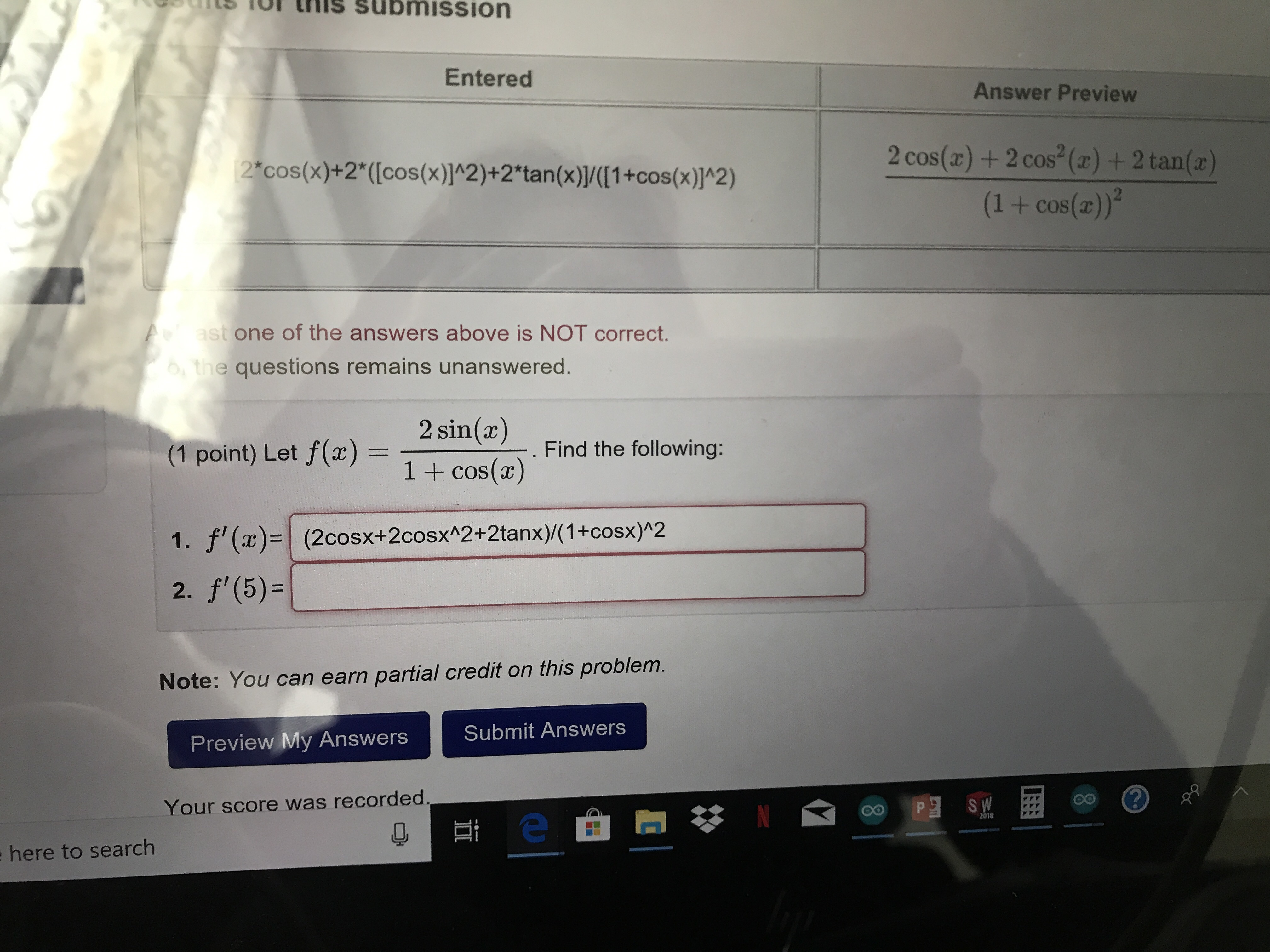# tTthisSubmissionEnteredAnswer Preview2 cos() +2 cos(z) + 2 tan()(1 + cos(a))2cos(x)+2((cos(x)]A2)+2"tan(x)(1+cos(x)]*2)one of the answers above is NOT correct.the questions remains unanswered.2 sin(z)(1 point) Let f(z) = 1 + cos(x)Find the following:. f(2cosx+2cosxA2+2tanx) (1+cosx)h22.Note: You can earn partial credit on this problem.Submit AnswersPreview My AnswersYour score was recordedOoP2018here to search

Question
5 viewshelp_outlineImage Transcriptioncloset T this Submission Entered Answer Preview 2 cos() +2 cos(z) + 2 tan() (1 + cos(a)) 2cos(x)+2((cos(x)]A2)+2"tan(x)(1+cos(x)]*2) one of the answers above is NOT correct. the questions remains unanswered. 2 sin(z) (1 point) Let f(z) = 1 + cos(x) Find the following: . f(2cosx+2cosxA2+2tanx) (1+cosx)h2 2. Note: You can earn partial credit on this problem. Submit Answers Preview My Answers Your score was recorded OoP 2018 here to search fullscreen
check_circle

Step 1

(1)

We will find derivative

We can use quotient rule

Step 2

Quotient rule formula is

Step 3

Now, we can compare and find u(...

### Want to see the full answer?

See Solution

#### Want to see this answer and more?

Solutions are written by subject experts who are available 24/7. Questions are typically answered within 1 hour.*

See Solution
*Response times may vary by subject and question.
Tagged in

### Calculus